## Conditional probability: why is it defined like that?

So, you want to calculate the probability of an event knowing that another has happened. There is a formula for that, it is called conditional probability, but why is it the way it is? Let’s first write down the definition of conditional probability:We need to wonder: what does the happening of event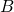tell about the odds of happening of event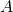? How much more likelybecomes ifhappens? Think in terms of howaffects.

Ifandare independent, then knowing something about B will not tell us anything at all about, at least not that we did not know already. In this caseis empty and thus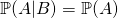. This makes sense! In fact, consider this example: how does me buying a copybook affects the likelihood that your grandma is going to buy a frying pan? It does not: the first event has no influence on the second, thus the conditional probability is just the same as the normal probability of the first event.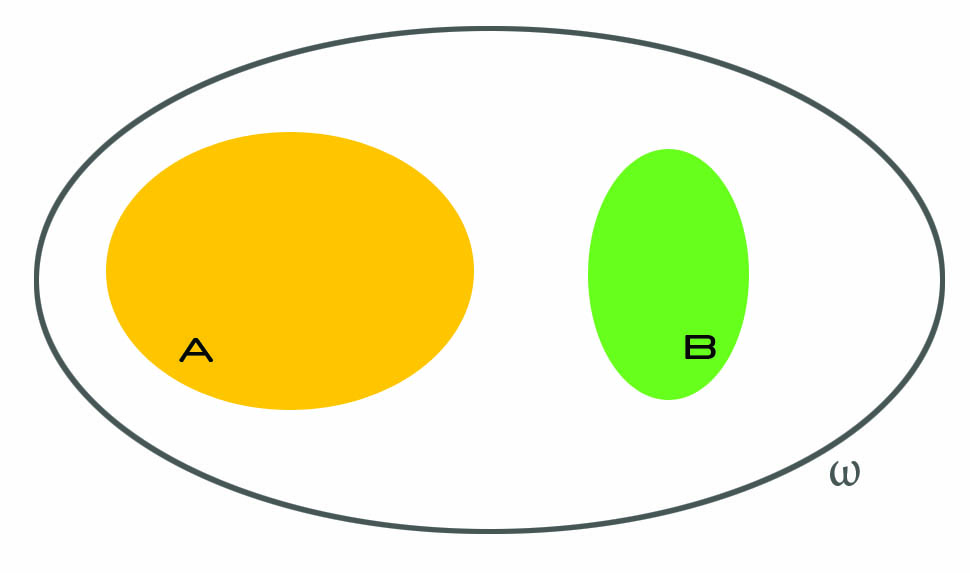Ifandare not independent, several things can happen, and that is where things get interesting. We know that B happened, and we should now think as ifwas our whole universe. The idea is: we already know what are the odds of, right? It is just. But how do they increase if we know that we do not really have to consider all possible events, but just a subset of them? As an example, think of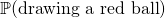versusknowing that all balls are red. This makes a huge difference, right? (As an aside, that is what we mean when we say that probability is a measure of our ignorance.)

So anyway, now we ask: what is the probability of? Well, it would just be, but we must account for the fact that we now live inside, and everything that is outside it is as if it did not existed. Soactually becomes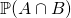: we only care about the part ofthat is inside, because that is where we live now.

But, there is a caveat. Continue reading “Conditional probability: why is it defined like that?”

## Diagonalizing a matrix NOT having full rank: what does it mean?

This is going to be a quick intuition about what it means to diagonalize a matrix that does not have full rank (i.e. has null determinant).

Every matrix can be seen as a linear map between vector spaces. Stating that a matrix is similar to a diagonal matrix equals to stating that there exists a basis of the source vector space in which the linear transformation can be seen as a simple stretching of the space, as re-scaling the space. In other words, diagonalizing a matrix is the same as finding an orthogonal grid that is transformed in another orthogonal grid. I recommend this article from AMS for good visual representations of the topic.

### Diagonalization on non full rank matrices

That’s all right – when we have a matrix from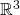in, if it can be diagonalized, we can find a basis in which the transformation is a re-scaling of the space, fine.

But what does it mean to diagonalize a matrix that has null determinant? The associated transformations have the effect of killing at least one dimension: indeed, axmatrix of rank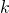has the effect of lowering the output dimension by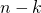. For example, a 3×3 matrix of rank 2 will have an image of size 2, instead of 3. This happens because two basis vectors are merged in the same vector in the output, so one dimension is bound to collapse.

Let’s consider the sample matrix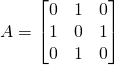which has non full rank because has two equal rows. Indeed, one can check that the two vectorsgo in the same basis vector. This means thatinstead of 3. In fact, it is common intuition that when the rank is not full, some dimensions are lost in the transformation. Even if it’s a 3×3 matrix, the output only has 2 dimensions. It’s like at the end of Interstellar when the 4D space in which Cooper is floating gets shut.

However,is also a symmetric matrix, so from the spectral theorem we know that it can be diagonalized. And now to the vital questions: what do we expect? What meaning does it have? Do we expect a basis of three vectors even if the map destroys one dimension?

Pause and ponder.

## Finding paths of length n in a graph

Suppose you have a non-directed graph, represented through its adjacency matrix. How would you discover how many paths of lengthlink any two nodes?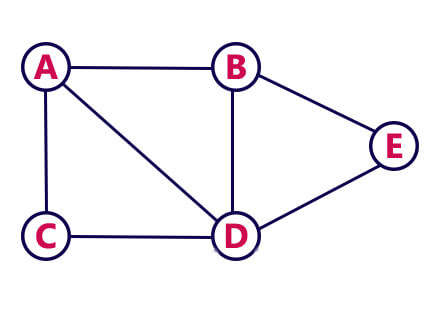For example, in the graph aside there is one path of length 2 that links nodes A and B (A-D-B). How can this be discovered from its adjacency matrix?

It turns out there is a beautiful mathematical way of obtaining this information! Although this is not the way it is used in practice, it is still very nice. In fact, Breadth First Search is used to find paths of any length given a starting node.

PROP.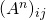holds the number of paths of lengthfrom nodeto node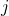.

Let’s see how this proposition works. Consider the adjacency matrix of the graph above:With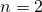we should find paths of length 2. So we first need to square the adjacency matrix:

## On the relationship between L^p spaces and C_c functions for p = infinity

Very quick post on the relationship between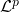,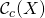and. I will assume you already know what I am talking about, I’ll just be sharing some intuition on what those mean, but won’t bother with details. It’s more a reminder for me rather than something that intends to be useful, actually, but there’s almost nothing on the Internet about this!

When we discover that(continuous functions with compact support) is dense in, we also discover that it does not hold if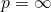and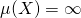.

What that intuitively means is that if you take away functions infrom, you take away something fundamental for: you are somehow taking away a net that keeps the ceiling up.

The fact that it becomes false for limitless spaces () andmeans that the functions indo not need functions into survive.

This is reasonable: functions inare not required to exist only in a specific (compact) region of space, whereas functions indo. Functions inare simply bounded – their image keeps below some value, but can go however far they want in x direction. Very roughly speaking, they have a limit on their height, but not on their width.

What we find out, however, is that the following chain of inclusions holds: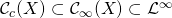## The meaning of F Value in the Analysis of Variance for Linear regression

This is a sample output for linear regression: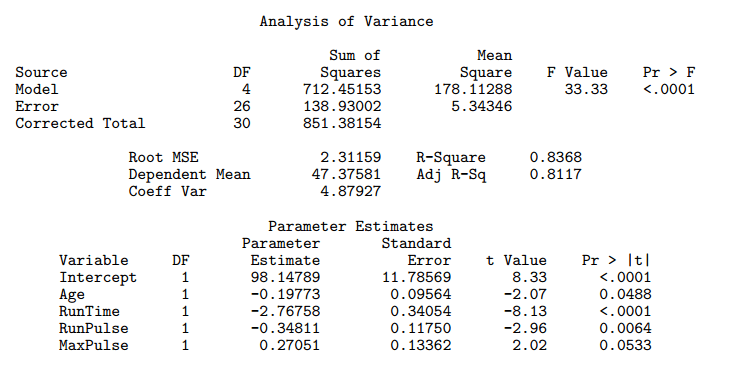The F Value is computed by dividing the value in the Mean Square column for Model with the value in the Mean Square column for Error. In our example, it’s.

There are two possible interpretations for the F Value in the Analysis of Variance table for the linear regression.

## On the meaning of hypothesis and p-value in statistical hypothesis testing

Statistical hypothesis testing is really an interesting topic. I’ll just briefly sum up what statistical hypothesis testing is about, and what you do to test an hypothesis, but will assume you are already familiar with it, so that I can quickly cover a couple of A-HAs moments I had.

In statistical hypothesis testing, we

• have some data, whatever it is, which we imagine as being values of some random variable;
• make an hypothesis about the data, such as that the expected value of the random variable is;
• find a distribution for any affine transformation of the random variable we are making inference about – this is the test statistic;
• run the test, i.e. numerically say how much probable how observations were in relation to the hypothesis we made.

I had a couple of A-HA moments I’d like to share.

There is a reason why this is called hypothesis testing and not hypothesis choice. There are indeed two hypothesis, the null and the alternative hypothesis. However, their roles are widely different! 90% of what we do, both from a conceptual and a numerical point of view, has to do with the null hypothesis. They really are not symmetric. The question we are asking is “With the data I have, am I certain enough my null hypothesis no longer stands?” not at all “With the data I have, which of the two hypothesis is better?”

## Why hash tables should use a prime-number size

I read in several books and online pages that hash tables should use a prime number for the size. Nobody really justified this statement properly. Here’s my attempt!

I believe that it just has to do with the fact that computers work with in base 2. Just think at how the same thing works for base 10:

• 8 % 10 = 8
• 18 % 10 = 8
• 87865378 % 10 = 8
• 2387762348 % 10 = 8

It doesn’t matter what the number is: as long as it ends with 8, its modulo 10 will be 8. You could pick a huge power of 10 as modulo operator, such as 10^k (with k > 10, let’s say), but

1. you would need a huge table to store the values
2. the hash function is still pretty stupid: it just trims the number retaining only the first k digits starting from the right.

However, if you pick a different number as modulo operator, such as 12, then things are different:

• 8 % 12 = 8
• 18 % 12 = 6
• 87865378 % 12 = 10
• 2387762348 % 12 = 8

We still have a collision, but the pattern becomes more complicated, and the collision is just due to the fact that 12 is still a small number.

Picking a big enough, non-power-of-two number will make sure the hash function really is a function of all the input bits, rather than a subset of them.

## Metaphysics on geometric distribution in probability theory

I realized geometric distribution is not exactly about the time needed to get the first success in a given number of trials. This is a very odd feeling. It is probably a feeling applied mathematicians get sometimes, when they feel they are doing the best they can, and yet the theory is not perfect.

This may be a naive post, I warn you, but I was really stunned when I realized this.

### Geometric distribution is not about the first success

Let’s jump to the point. We know (or at least, I was taught) that geometric distribution is used to calculate the probability that the first success intrials (all independent and of probability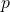) will happen precisely at the-th trial.

Remember that a geometric distribution is a random variablesuch that its distribution is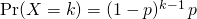How can we relate the above distribution with the fact that it matches the first success? Well, we need to have one success, which explains theat the bottom. Moreover, we want to have just one success, so all other trials must be unsuccessful, which explains the.

But hey, where would first ever be written? Continue reading “Metaphysics on geometric distribution in probability theory”

## Random variables: what are they and why are they needed?

This article aims at providing some intuition for what random variables are and why random variables are useful and needed in probability theory.

## Intuition for random variables

Informally speaking, random variables encode questions about the world in a numerical way.

How many heads can I get if I flip a coin 3 times?

How many people will vote the Democrats at the US presidential elections?

I want to make pizza. What is the possible overall cost of the ingredients, considering all combinations of different brands of them?

These are all examples of random variables. What a random variable does, in plain words, is to take a set of possible world configurations and group them to a number. What I mean when I say world configurations will be clearer soon, when talking about the sample space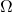(which, appropriately, is also called universe).

## Relationship between reduced rings, radical ideals and nilpotent elements

This post aims at providing some intuition and meaning for the following algebra relationship:

Reduced ring – Radical ideal – Nilpotent

## Reduced ring – Radical ideal – Nilpotent

A basic fact of ring theory is that if you take a ringand quotient it for a (double-sided) radical idealyou get a reduced ring. Let us suppose A is a commutative ring and understand why this fact is true.

Nilpotent element
Def.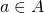is nilpotentInformally, a nilpotent element is like a road ending in the middle of nowhere, collapsing in the depth of an abyss. You are driving on it, following the powers of, and then all of a sudden, with no explanation, your road ends in a big black hole. Indeed, the zero really acts as some kind of black hole, attracting nilpotent-made roads at some point or another: we can think of nilpotent roads as spiraling into the zero.